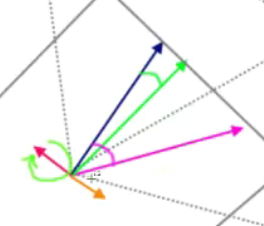# Rotating a vector

So why don’t just change it by a fraction, are using a RunService event to update it?

Why not use body movers to smoothly move your projectile? You could use `BodyPosition` or ` RocketPropulsion`.

I don’t think you understand what you need. Use TweenService to tween (smoothly interpolate) the projectile to be in the direction of the mouse. That is what that service is for.

Edit: Otherwise you need to use a rotation matrix - you can’t rotate a 3D vector to another 3D vector by itself without a matrix. This is accomplished with CFrame.new(pos, targetPos)

Although using runservice is very fast its not a reliable method since there no gunatureed method it will clamp between desiredangle,maxangle… as for the fraction there be wasted movement since if you can imaigne 2 vectors being very close to each together (small angle) then it will have a hard time reaching to that point since it would then be a small number

Alright Ill try the matrix method and get back to you on that then

Yes, but don’t ignore TweenService. You need to use it so it doesn’t “snap” to the new position. There’s a reason I keep telling you about it - I’m not just randomly throwing it out there.

Use a tween with CFrame.new(pos, targetPos) to tween the projectile to face the new mouse direction (rotate the vector).

``````local projectile = ? -- Your rocket or whatever
local ts = game:GetService("TweenService")
local tweenInfo = TweenInfo.new(1) -- 1 is the time of interpolation for the tween
local tween = ts:Create(projectile, tweenInfo, {
CFrame = CFrame.new(projectile.Position, mouse.Hit.p)
})

tween:Play() -- Run the tween
``````

Does tweenservice have any issues with the physics engine, the projectile im working with is being moved by bodyvelocity so it is therefor unanchored

Ah, I see. Then you need to use BodyGyro and set the CFrame of that instead of TweenService.

1 Like

Dot Product is telling you how close one vector is to pointing towards the other. If the Dot Product is 1 then both vectors are in the same direction. -1 means they are both facing opposite sides. 0 or -0 means they are perpendicular to each other.

A little confused by the question can you further elaborate on what you mean by controlling the projectile with the player mouse. Specifically how that relates to needing to rotate a vector?

2 Likes

As an addition to @EgoMoose’s question, could you also explain your diagrams as its difficult to see what your end goal is when you then proceed to talk about the mouse controlling the projectile (the diagrams don’t relate)?

I’m assuming you want a projectile which you can fire, and then your mouse can influence how it moves through the air?

1 Like

Yes the projectile velocity changes based on the mouse

I want my projectile to steer towards my mouse instead of instant

If it’s physics simulated, you have to apply a force correcting the path, which is usually perpendicular to the velocity.

``````local planeN = mouseDir.Unit:Cross(velocity.Unit)
local sideVec = velocity.Unit:Cross(planeN)
VectorForce.RelativeTo = Enum.ActuatorRelativeTo.World
VectorForce.Force = k * sideVec --  k being a proportional multiplier
``````

Can you link something showing this? Perhaps another game etc? I’m still not understanding.

``````function rotateVectorAround( v, amount, axis )
return CFrame.fromAxisAngle(axis, amount):VectorToWorldSpace(v)
end
``````

Example use:

Script
``````local VERTICAL = Vector3.new(0, 1, 0)
local HORIZONTAL = Vector3.new(1, 0, 1)

local rotateSpeed = 1
local followSpeed = 20

local bullet = script.Parent.Bullet
local target = script.Parent.Target

function rotateVectorAround( v, amount, axis )
return CFrame.fromAxisAngle(axis, amount):VectorToWorldSpace(v)
end

--Skip the first heartbeat because it's dt is too high because it waits for game to load
game:GetService("RunService").Heartbeat:Wait()

game:GetService("RunService").Heartbeat:Connect(function(dt)
local dirToTarget = bullet.CFrame:VectorToObjectSpace((target.Position - bullet.Position))
local angleToTarget = math.atan2(-dirToTarget.X, -dirToTarget.Z)
local rotateDir = (angleToTarget < 0 and -1) or (angleToTarget > 0 and 1) or (0)
local rotateAmount = math.clamp(rotateDir * rotateSpeed * dt, -math.abs(angleToTarget), math.abs(angleToTarget))

bullet.CFrame = CFrame.new(
bullet.Position,
bullet.Position + rotateVectorAround(bullet.CFrame.LookVector, rotateAmount, VERTICAL)
) * CFrame.new( Vector3.FromNormalId(Enum.NormalId.Front) * followSpeed * dt )
end)
``````

Test Model (3.5 KB)

18 Likes

I believe what hes trying to do is create a bullet which upon being fired can alter its trajectory and curve towards the mouse’s current position. So upon firing the bullets, the bullet can then be guided by the cursor towards its target much like how a laser guidance works in real life.

I do not however, have much experience in this. But on a physics basis, it would be applying a counter force (with a maximum force I assume so that it doesn’t just immediately switch) in order to correct the current trajectory towards the new trajectory. He hasn’t however, specified how he wants the trajectory to be corrected.

https://gyazo.com/f3239662891f0cf2c57a41e3503fef3e.mp4

here the actual game im using it for, it kinda works right now but due to my current code it doesn’t work well when shooting directly at something

My current solution im trying to do (at this moment) is to cross product the velocity vector and mouseposition vector to get a perpdiencular vector and then rotating that matrix with cframe axisangle to get the new velocity vector

blue vector = velocity
green vector = desired velocity
pink vector = mouseposition
red vector = cross product vectorEdited : just saw @ThanksRoBama solution and that is esstinally what I believe my solution is what I am trying

2 Likes

Yes that is what I’m essentially trying to do

I did it a bit different from the way you did it but I still used your function``````local Step = wait()
local MouseOrigin = Mouse.UnitRay.Origin
local MouseDirection = Mouse.UnitRay.Direction
local MouseProjection = MouseOrigin + MouseDirection*(MouseDirection:Dot(Part.Position-MouseOrigin) + Data.Speed*Step*4)
local CrossVector = PartVelocity:Cross(MouseProjection-Part.Position)
local UnitCross = CrossVector.Unit

if UnitCross == ZeroVector then continue end

local Angle = math.acos(PartVelocity.Unit:Dot( (MouseProjection-Part.Position).Unit ))
if Angle ~= Angle then continue end

local RotateAmount = math.min(RotateSpeed*Step,Angle)

local NewVelocity = RotateVectorAround(BodyVelocity.Velocity/Data.Speed,RotateAmount,UnitCross)

BodyVelocity.Velocity = NewVelocity.Unit*Data.Speed
PartVelocity = BodyVelocity.Velocity
``````

Thank you everyone for the suggestions2 Likes